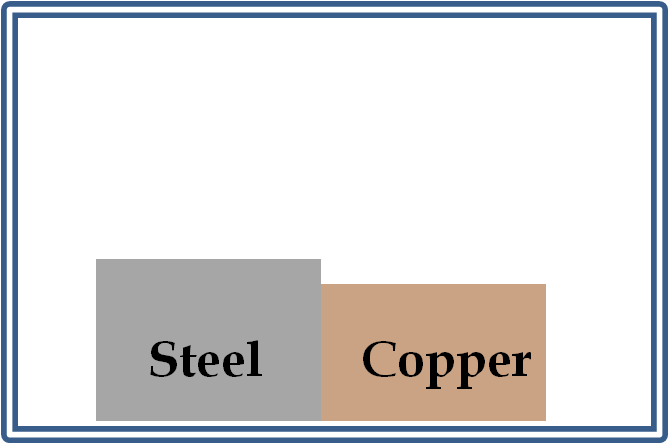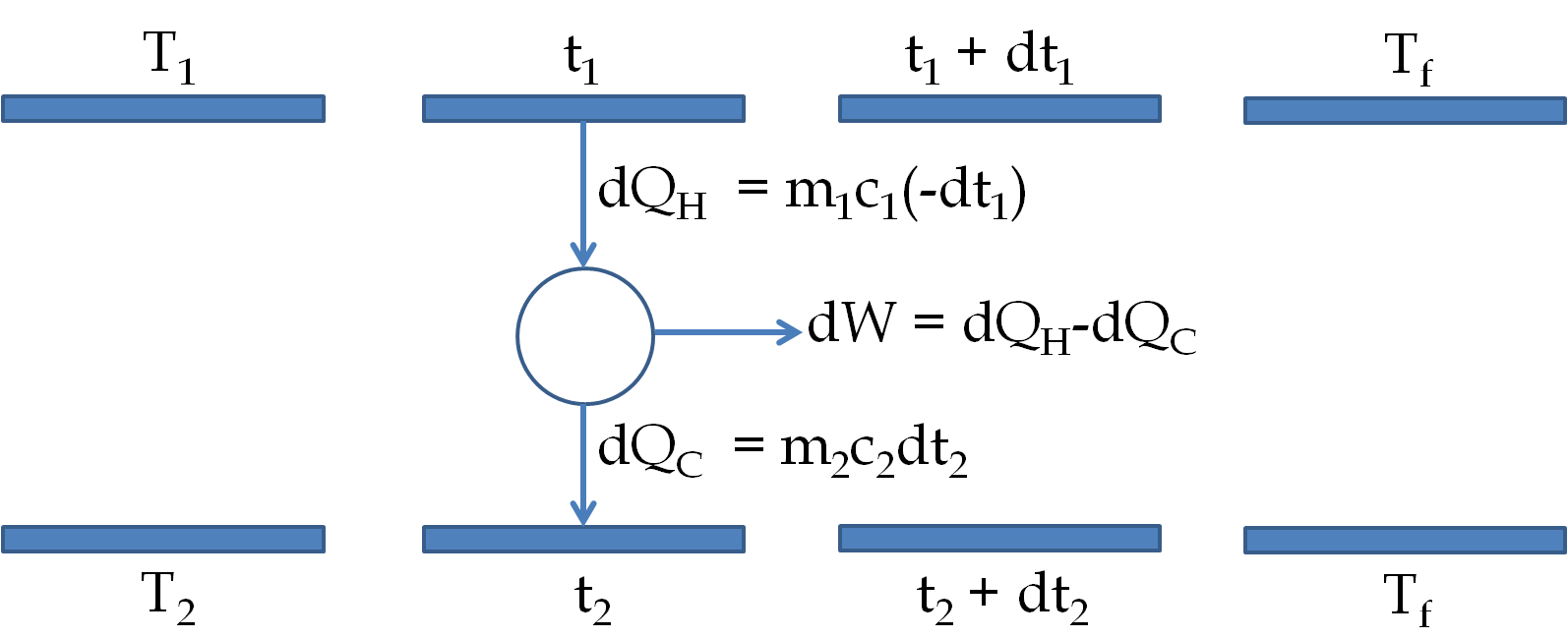## Section26.9Entropy Bootcamp

### Subsection26.9.3Miscellaneous

A 100-g ice cube at $-10^{\circ}$C is dropped into 500 g of liquid water at $60^{\circ}$C in a glass beaker of mass 400 g in a thermally insulated environment. (a) Find the final state of the ice/water mixture. (b) Calculate the change in the entropy from the initial state to the final state.

Data: $c_{i} = 2000\ \text{J/kg.C}\text{,}$ $c_{w} = 4186 \ \text{J/kg.C} \text{,}$ $c_{g} = 837 \ \text{J/kg.C} \text{,}$ $l = 334,000 \ \text{J/kg}\text{.}$

Hint

(a) Use calorimetry, (b) $\Delta S = \dfrac{ml}{T}$ for phase change and $\Delta S = m c \ln \frac{T_2}{T_1}$ for change in temperature.

(a) See solution (a), (b) $25 \ \text{J/K}\text{.}$

Solution 1 (a)

(a) For a more detailed discussion of calorimetry see problems in the chapter on the first law of thermodynamics. Suffice to say that the energy lost by water and the beaker is balanced by the energy gained by the ice.

A rough calculation shows that all ice melts and the temperature of the mixture will be more than 0$^{\circ}$C. Let $T$ be the temperature at the equilibrium. Then, the equation balancing the energy gained to energy lost gives.

\begin{align*} \amp 0.1\ \text{kg} \left( 2000\ \text{J/kg.C} \times 10^{\circ}\text{C} + 334,000 \ \text{J/kg} + 4186\ \text{J/kg.C} \times T \right)\\ \amp \ \ = ( 0.5\ \text{kg} \times 4186\ \text{J/kg.C} + 0.4\ \text{kg} \times 837\ \text{J/kg.C} )\times ( 60 - T). \end{align*}

Solving this equation we find $T = 38.7^{\circ}$C. Therefore, the change of state can be described as

\begin{align*} \amp \text{Initial State: }\\ \amp \ \ \ \ \ \ \ \text{1. 0.1 kg ice at -10}^{\circ}\text{C}\\ \amp \ \ \ \ \ \ \ \text{2. 0.5 kg of water at 60}^{\circ}\text{C}\\ \amp \ \ \ \ \ \ \ \text{3. 0.4 kg of glass at 60}^{\circ}\text{C} \end{align*}
\begin{align*} \amp \text{Final State: }\\ \amp \ \ \ \ \ \ \ \text{1. 0.1 kg water at 38.7}^{\circ}\text{C}\\ \amp \ \ \ \ \ \ \ \text{2. 0.5 kg of water at 38.7}^{\circ}\text{C}\\ \amp \ \ \ \ \ \ \ \text{3. 0.4 kg of glass at 38.7}^{\circ}\text{C} \end{align*}
Solution 2 (b)

(b) For each subsystem we can calculate the entropy change by infinitesimal steps in temperature, except for the step where the phase changes. In the step of phase change the ratio of heat in to temperature will be accompanied by no change in the temperature. The formula for the entropy change when a change in temperature occurs is

\begin{equation*} \Delta S = m\,c\,\ln\dfrac{T_2}{T_1}. \end{equation*}

We will just write the answer for the changes in entropy for each subsystem here.

\begin{align*} \amp \Delta S_{ice} = m_i c_i \ln\left(\frac{0+273}{-10+273} \right) + \frac{m_i l}{0+273} \\ \amp \ \ \ \ \ \ \ \ \ \ \ \ \ \ + m_i c_w \ln\left(\frac{38.7+273}{0+273} \right) = 185\ \text{J/K}. \end{align*}
\begin{align*} \amp \Delta S_{w} = m_w c_w \ln\left(\frac{38.7+273}{60+273} \right) = -138\ \text{J/K}.\\ \amp \Delta S_{g} =m_g c_g \ln\left(\frac{38.7+273}{60+273} \right)=-22\ \text{J/K}. \end{align*}

Summing up the changes in the subsystems we find the net change of entropy from the initial state to the final state.

\begin{equation*} \Delta S = \Delta S_{ice} + \Delta S_{w} + \Delta S_{g} = 25 \ \text{J/K}. \end{equation*}

A $50\text{-kg}$ copper block at $400 \text{ K}$ is placed in contact with a $75\text{-kg}$ steel block at $300 \text{ K}$ in an insulated environment from other objects as shown in the figure.(a) Find the final temperature of the blocks.

(b) Find the change in the internal energy of each block.

(c) (Calculus) Find the change in the entropy of each block. For Calculus-based course integrate $dS = m\,c\,dT/T\text{.}$ For non-Calculus, use formula $\Delta S = m\,c\,\ln(T_2/T_1)\text{.}$

(d) Find the total entropy change.

(e) Why is the total entropy change not equal to zero even when no heat is exchanged with the environment?

Hint

(a) Use calorimetry concepts, (b) Use $mc\Delta T\text{,}$ (c) integrate $m\,c\,dT/T$ or use formula $m\,c\,\ln(T_2/T_1)\text{,}$ (d) Just use the results from (c), (e) Think reversible versus irreversible process.

(a) $334\ \text{K}\text{,}$ (b) $-1.3\ \text{MJ}\text{,}$ $+1.3\ \text{MJ} \text{,}$ (c) $-3,500 \ \text{J/K} \text{,}$ $4,000 \ \text{J/K}\text{,}$ (d) $500 \ \text{J/K} \text{,}$ (e) Irreversible process.

Solution 1 (a)

(a) For the sake of simplicity in presentation let us denote the quantities pertaining to the copper block by subscript 1 and those to the steel block by the subscript 2.

The volume change of the blocks will be very small. These expansions occur against atmospheric pressure, making the work negligible compared to the heat. Therefore, from the first law we note that the change in the internal energy of each block is accounted for by the heat flow only.

\begin{equation*} \Delta U_{1} \approx Q_{\text{into }1}. \end{equation*}

Since no change of phase takes place we can write the $Q$ in terms of specific heat. Although specific heat is a function of temperature, we will assume that the specific heat does not change much so that

\begin{equation*} Q = m c \Delta T. \end{equation*}

Let $T_f$ be the final temperature of the two blocks. Then, the change in the energy of the copper block will be:

\begin{equation*} \Delta U_{1} = m_{1} c_{1} (T_f-T_1). \end{equation*}

Similarly, the change in the energy of the steel block will be

\begin{equation*} \Delta U_{2} = m_{2} c_{2} (T_f-T_2). \end{equation*}

The total energy of the two blocks does not change since the two blocks do not interact with any other systems. Therefore,

\begin{equation*} \Delta U_{1} + \Delta U_{2} = 0, \end{equation*}

which gives

\begin{equation*} m_{1} c_{1} (T_f-T_1) + m_{2} c_{2} (T_f-T_2) = 0. \end{equation*}

Solving for $T_f$ we get

\begin{equation*} T_f = \frac{m_{1} c_{1} T_1 + m_{2} c_{2} T_2 }{m_{1} c_{1} + m_{2} c_{2} }. \end{equation*}

Now, we put in the following numbers.

\begin{align*} \amp m_1 = 50\ \text{kg},\ \ c_1 = 390\ \text{J/kg.K}, \ \ T_1 = 400\ \text{K}\\ \amp m_2 = 75\ \text{kg},\ \ c_2 = 500 \ \text{J/kg.K}, \ \ T_2 = 300\ \text{K} \end{align*}

Therefore

\begin{align*} T_f \amp = \frac{50\ \text{kg}\times 390\ \text{J/kg.K}\times 400\ \text{K} + 75\ \text{kg}\times 500 \ \text{J/kg.K}\times 300\ \text{K} }{50\ \text{kg}\times 390\ \text{J/kg.K} + 75\ \text{kg}\times 500 \ \text{J/kg.K} }\\ \amp = 334\ \text{K}. \end{align*}
Solution 2 (b)

(b) The change in internal energy of the blocks are

\begin{align*} \amp \Delta U_{1} = m_{1} c_{1} (T_f-T_1) = -1.3\ \text{MJ}.\\ \amp \Delta U_{2} = m_{2} c_{2} (T_f-T_2) = +1.3\ \text{MJ}. \end{align*}
Solution 3 (c)

(c) The change in the entropy of the copper block will be built from the formula for an infinitesimal change in the temperature of the block.

\begin{equation*} dS = \frac{dQ}{T} = \frac{mcdT}{T}. \end{equation*}

Since $c$'s of the blocks are constant we obtain

\begin{align*} \amp \Delta S_1 = m_1c_1\ln\left( \frac{T_f}{T_1}\right).\\ \amp \Delta S_2 = m_2c_2\ln\left( \frac{T_f}{T_2}\right). \end{align*}

Putting in the numbers we get

\begin{align*} \amp \Delta S_1 = -3,500 \ \text{J/K}.\\ \amp \Delta S_2 = +4,000\ \text{J/K}. \end{align*}
Solution 4 (d)

(d) The total entropy change $= (4,000 - 3500)\text{ J/K} = 500 \text{ J/K}\text{.}$

Solution 5 (e)

(e) The total change in the entropy of the copper and the steel blocks is not zero since the process from the initial state to the final state is an irreversible process. The copper block was initially at a higher temperature and the steel block at a lower temperature. Spontaneous process leads to heat loss from the higher temperature body, which corresponds to less reduction in the entropy of the higher-temperature body compared to the increase in the entropy of the lower temperature-body, which gains heat at a lower temperature.

Two water baths of mass 1000 kg each are to serve as heat source and heat sink for a Carnot engine. One of the baths is at temperature $T_1$ and the other at temperature $T_2$ with $T_1>T_2\text{.}$

In each cycle the Carnot engine takes an infinitesimal amount of heat $dQ_1$ from the higher temperature bath and produces an infinitesimal work $dW$ and rejects the remainder $dQ_2$ to the lower temperature reservoir.

As a result, the temperature of the higher temperature reservoir decreases in time while the temperature of the lower temperature bath increases until the two baths reach the same final temperature $T_f$ at which point the engine stops producing any work.

(a) Find $T_f$ in terms of the initial temperatures $T_1$ and $T_2$ of the two baths.

(b) Evaluate the total work produced.

(c) Find the net change in the entropy.

Hint

(a) Examine an infinitesimal process in which temperatures change a little bit - in this step use the Carnot engine relation between heats transfered and absolute temperatures. (b) Set up work in an infinitesimal step, (c) Add up changes in the entropy of the baths as they go from their initial temperatures to the final temperature.

(a) $T_f = \sqrt{T_1 T_2}\text{,}$ (b) $mc ( T_1 + T_2 - 2\sqrt{T_1T_2})\text{,}$ (c) 0.

Solution 1 (a)

(a) The two baths together with the Carnot engine between the them do not form an isolated system since the work produced by the Carnot engine is sent to an outside body. Therefore, the net energy of the three parts of the system is not conserved. To find the final temperature of the baths we will examine the infintesimal processes in which the changes in the temperature of the baths takes place as shown in the figure below.

Let the higher-temperature bath be at the temperature $t_1$ and the lower-temperature bath at the temperature $t_2\text{.}$ Consider infinitesimal changes in the two and the work $dW$ produced during the infinitesimal process shown in the figure.Since the engine between the baths is a Carnot engine the heat exchanged from the baths are proportional to their temperatures in the absolute scale.

\begin{equation*} \frac{m_1 c_1 (-dt_1)}{m_2 c_2 dt_2} = \frac{t_1}{t_2}, \end{equation*}

where the negative sign is included since $dt_1 \lt 0$ and all other quantities are positive here. Separating the variables and integrating from the initial temperatures $T_1$ and $T_2$ to $t_1$ and $t_2$ we obtain

\begin{equation*} m_1 c_1 \ln\left( \frac{T_1}{t_1}\right) = m_2 c_2 \ln\left( \frac{t_2}{T_2}\right). \end{equation*}

Rather than work with the general case, we specialize to the present case of $m_1=m_2$ and $c_1 = c_2$ to obtain

\begin{equation*} \ln\left( \frac{T_1}{t_1}\right) = \ln\left( \frac{t_2}{T_2}\right). \end{equation*}

This can be written as

\begin{equation} t_1 t_2 = T_1 T_2.\label{}\label{eq-ch20-pr-4-1}\tag{26.9.1} \end{equation}

For part (a), we apply this to the final temperature $T_f$ of the two baths we obtain

\begin{equation*} T_f = \sqrt{T_1 T_2} \end{equation*}

We will use Eq. (26.9.1) to write $t_2$ in terms of $t_1$ in the following.

Solution 2 (b)

(b) Now, the work in the infinitesimal step is given by

\begin{equation*} dW = dQ_H - dQ_C = mc\left[ (-dt_1) - dt_2 \right] \end{equation*}

Now using Eq. (26.9.1) we write this as

\begin{equation*} dW = mc\left[ (-dt_1) +\frac{T_1T_2}{t_1^2} dt_1\right]. \end{equation*}

We can now integrate from $t_1 = T_1$ to $t_1= T_f$ to obtain the total work produced.

\begin{align*} W \amp = m c ( T_1 - T_f) - mcT_1T_2 \left[ \frac{1}{T_f} - \frac{1}{T_1}\right]\\ \amp = mc ( T_1 + T_2 - 2\sqrt{T_1T_2}). \end{align*}
Solution 3 (c)

(c) The entropy change of the Carnot engine is zero but for the materials of the baths may not be zero. Let us calculate it out and see what we get. The calculations for the baths will go similar to the copper and steel blocks worked out above.

\begin{align*} \amp \Delta S_1 = m c \ln \left( \frac{T_f}{T_1}\right).\\ \amp \Delta S_2 = m c \ln \left( \frac{T_f}{T_2}\right). \end{align*}

Adding the two we get the net change in entropy

\begin{equation*} \Delta S = m c \ln \left( \frac{T_f^2}{T_1T_2}\right) = m c \ln \left( 1\right) = 0. \end{equation*}

Since all processes in the given system occur infinitesimally and reversibly, the net entropy change is zero.